# `sklearn.cluster`.MiniBatchKMeans¶

class `sklearn.cluster.``MiniBatchKMeans`(n_clusters=8, init=’k-means++’, max_iter=100, batch_size=100, verbose=0, compute_labels=True, random_state=None, tol=0.0, max_no_improvement=10, init_size=None, n_init=3, reassignment_ratio=0.01)[source]

Mini-Batch K-Means clustering

Read more in the User Guide.

Parameters: n_clusters : int, optional, default: 8 The number of clusters to form as well as the number of centroids to generate. init : {‘k-means++’, ‘random’ or an ndarray}, default: ‘k-means++’ Method for initialization, defaults to ‘k-means++’: ‘k-means++’ : selects initial cluster centers for k-mean clustering in a smart way to speed up convergence. See section Notes in k_init for more details. ‘random’: choose k observations (rows) at random from data for the initial centroids. If an ndarray is passed, it should be of shape (n_clusters, n_features) and gives the initial centers. max_iter : int, optional Maximum number of iterations over the complete dataset before stopping independently of any early stopping criterion heuristics. batch_size : int, optional, default: 100 Size of the mini batches. verbose : boolean, optional Verbosity mode. compute_labels : boolean, default=True Compute label assignment and inertia for the complete dataset once the minibatch optimization has converged in fit. random_state : int, RandomState instance or None, optional, default: None If int, random_state is the seed used by the random number generator; If RandomState instance, random_state is the random number generator; If None, the random number generator is the RandomState instance used by np.random. tol : float, default: 0.0 Control early stopping based on the relative center changes as measured by a smoothed, variance-normalized of the mean center squared position changes. This early stopping heuristics is closer to the one used for the batch variant of the algorithms but induces a slight computational and memory overhead over the inertia heuristic. To disable convergence detection based on normalized center change, set tol to 0.0 (default). max_no_improvement : int, default: 10 Control early stopping based on the consecutive number of mini batches that does not yield an improvement on the smoothed inertia. To disable convergence detection based on inertia, set max_no_improvement to None. init_size : int, optional, default: 3 * batch_size Number of samples to randomly sample for speeding up the initialization (sometimes at the expense of accuracy): the only algorithm is initialized by running a batch KMeans on a random subset of the data. This needs to be larger than n_clusters. n_init : int, default=3 Number of random initializations that are tried. In contrast to KMeans, the algorithm is only run once, using the best of the `n_init` initializations as measured by inertia. reassignment_ratio : float, default: 0.01 Control the fraction of the maximum number of counts for a center to be reassigned. A higher value means that low count centers are more easily reassigned, which means that the model will take longer to converge, but should converge in a better clustering. cluster_centers_ : array, [n_clusters, n_features] Coordinates of cluster centers labels_ : : Labels of each point (if compute_labels is set to True). inertia_ : float The value of the inertia criterion associated with the chosen partition (if compute_labels is set to True). The inertia is defined as the sum of square distances of samples to their nearest neighbor.

`KMeans`
The classic implementation of the clustering method based on the Lloyd’s algorithm. It consumes the whole set of input data at each iteration.

Notes

Methods

 `fit`(X[, y]) Compute the centroids on X by chunking it into mini-batches. `fit_predict`(X[, y]) Compute cluster centers and predict cluster index for each sample. `fit_transform`(X[, y]) Compute clustering and transform X to cluster-distance space. `get_params`([deep]) Get parameters for this estimator. `partial_fit`(X[, y]) Update k means estimate on a single mini-batch X. `predict`(X) Predict the closest cluster each sample in X belongs to. `score`(X[, y]) Opposite of the value of X on the K-means objective. `set_params`(**params) Set the parameters of this estimator. `transform`(X) Transform X to a cluster-distance space.
`__init__`(n_clusters=8, init=’k-means++’, max_iter=100, batch_size=100, verbose=0, compute_labels=True, random_state=None, tol=0.0, max_no_improvement=10, init_size=None, n_init=3, reassignment_ratio=0.01)[source]
`fit`(X, y=None)[source]

Compute the centroids on X by chunking it into mini-batches.

Parameters: X : array-like or sparse matrix, shape=(n_samples, n_features) Training instances to cluster. y : Ignored
`fit_predict`(X, y=None)[source]

Compute cluster centers and predict cluster index for each sample.

Convenience method; equivalent to calling fit(X) followed by predict(X).

Parameters: X : {array-like, sparse matrix}, shape = [n_samples, n_features] New data to transform. u : Ignored labels : array, shape [n_samples,] Index of the cluster each sample belongs to.
`fit_transform`(X, y=None)[source]

Compute clustering and transform X to cluster-distance space.

Equivalent to fit(X).transform(X), but more efficiently implemented.

Parameters: X : {array-like, sparse matrix}, shape = [n_samples, n_features] New data to transform. y : Ignored X_new : array, shape [n_samples, k] X transformed in the new space.
`get_params`(deep=True)[source]

Get parameters for this estimator.

Parameters: deep : boolean, optional If True, will return the parameters for this estimator and contained subobjects that are estimators. params : mapping of string to any Parameter names mapped to their values.
`partial_fit`(X, y=None)[source]

Update k means estimate on a single mini-batch X.

Parameters: X : array-like, shape = [n_samples, n_features] Coordinates of the data points to cluster. y : Ignored
`predict`(X)[source]

Predict the closest cluster each sample in X belongs to.

In the vector quantization literature, cluster_centers_ is called the code book and each value returned by predict is the index of the closest code in the code book.

Parameters: X : {array-like, sparse matrix}, shape = [n_samples, n_features] New data to predict. labels : array, shape [n_samples,] Index of the cluster each sample belongs to.
`score`(X, y=None)[source]

Opposite of the value of X on the K-means objective.

Parameters: X : {array-like, sparse matrix}, shape = [n_samples, n_features] New data. y : Ignored score : float Opposite of the value of X on the K-means objective.
`set_params`(**params)[source]

Set the parameters of this estimator.

The method works on simple estimators as well as on nested objects (such as pipelines). The latter have parameters of the form `<component>__<parameter>` so that it’s possible to update each component of a nested object.

Returns: self :
`transform`(X)[source]

Transform X to a cluster-distance space.

In the new space, each dimension is the distance to the cluster centers. Note that even if X is sparse, the array returned by transform will typically be dense.

Parameters: X : {array-like, sparse matrix}, shape = [n_samples, n_features] New data to transform. X_new : array, shape [n_samples, k] X transformed in the new space.

## Examples using `sklearn.cluster.MiniBatchKMeans`¶Biclustering documents with the Spectral Co-clustering algorithm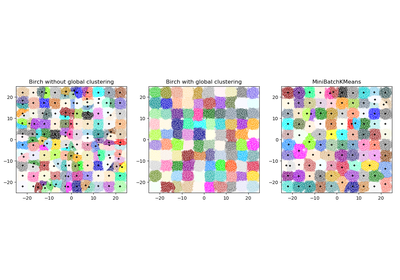Compare BIRCH and MiniBatchKMeans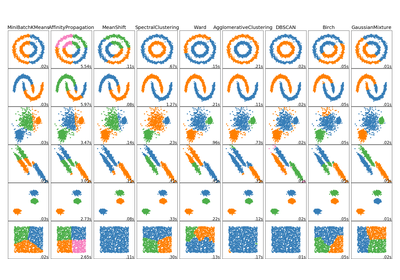Comparing different clustering algorithms on toy datasets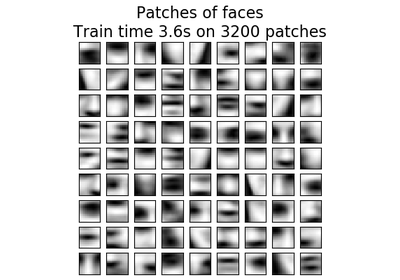Online learning of a dictionary of parts of faces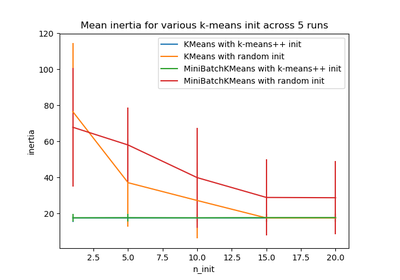Empirical evaluation of the impact of k-means initialization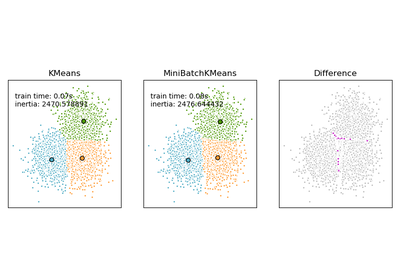Comparison of the K-Means and MiniBatchKMeans clustering algorithms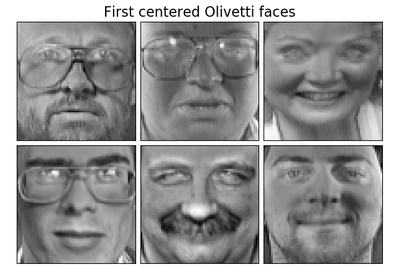Faces dataset decompositionsClustering text documents using k-means